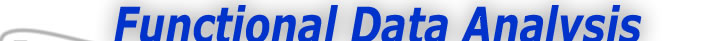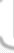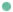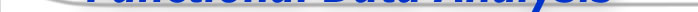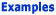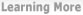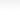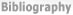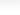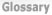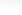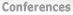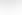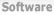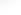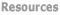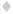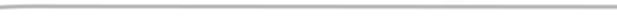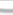BasisFunctions Goods Growth -What's wrong with fitting the data exactly? -How do I actually do this? -How do I actually do this? (cont.) Weather
 Smoothing the Growth Data Expertise: Beginner How do I actually do this? Here is a Matlab statement that smooths the height curves that we have already computed by penalizing the total curvature of the fourth derivative. hgtffd = smooth_fd(hgtffd, 0.01, int2Lfd(4)); The third argument specifies that it is the fourth derivative that we want to control. The second argument is a small constant that multiplies the roughness measure. The closer this constant is to zero, the less penalty is applied to roughness. The larger this constant is, the more roughness will be penalized, and ultimately we will have a curve with zero fourth derivative. Such a curve will be a third degree polynomial. Here we are applying a fairly light penalty to roughness. Figure 2 repeats Figure 1 of the previous example (See From Raw Data to Functional Data - Page 2). Perhaps at first we don't see much difference, and after all an error of about three millimeters is hard to see in a plot like this. But we do see in the title that the average residual is now 0.3 centimeters, which seems about right. In fact, this is how we chose the roughness penalty; we experimented with different values until we achieved this level of fit.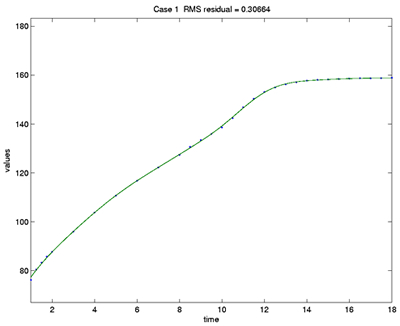Figure 2: The height of the first girl in the Berkeley Growth Study. Dots indicate the ages at which measurements were taken. The fitted curve has a typical error of about 0.3 centimeters, and is a result of penalizing roughness at the level of the fourth derivative.